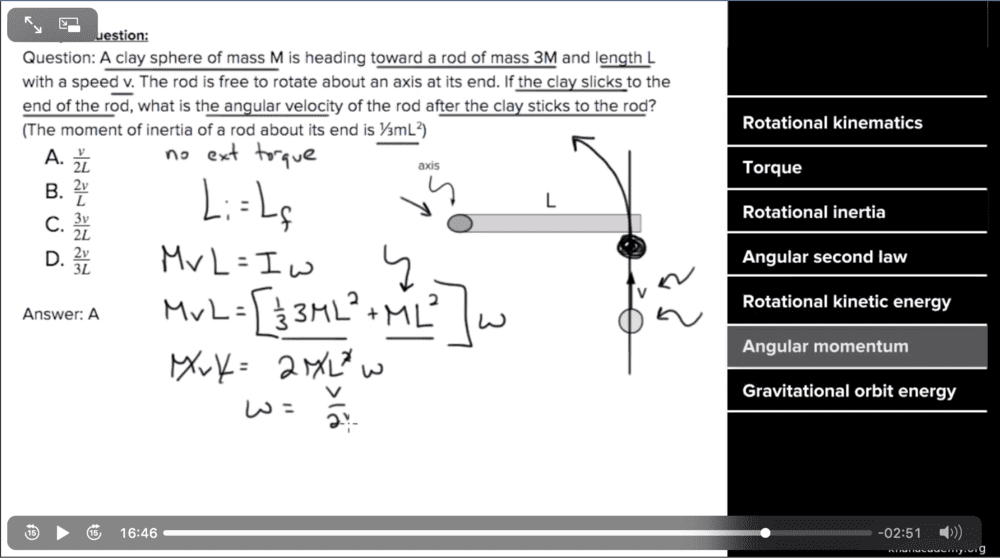# Angular Momentum in an Inelastic Collision

NP04
Homework Statement:
See image.
Relevant Equations:
Moment of inertia of end rod = 1/3ml^2I was wondering why in the video the moment of inertia for the clay ball (upon collision) was simply 1ml^2. That is the constant for a hollow cylinder. The problem specifies that the object is a ball, so the cylinder classification makes no sense, and also I'm pretty sure clay is rather dense of a material.

Shouldn't it instead be 2/5ml^2? That is the constant for a solid sphere through its center.
Thank you.

## Answers and Replies

Homework Helper
1ml^2
No. ML2, with L the length of the rod. Lower case m does not occur in the exercise. Nor does lower case l.
Please type your problem statement (see guidelines)

The ball doesn' t rotate around its center for this exercise: it rotates around the given axis and is considered to be a point mass.

Homework Helper
Gold Member
I was wondering why in the video the moment of inertia for the clay ball (upon collision) was simply 1ml^2.

Google "Moment of inertia of a particle about an axis". You will find it is equal to mr^2 or ml^2 depending if they use r or l for the tangential distance from the axis.

It's worth understanding how the moment of inertia for any shape can be calculated. It's effectively done by:

1) dividing the object into lots of individual small particles
2) calculating the moment of inertia for each particle
3) Adding up all the individual moments of inertia to give the total moment of inertia for the object.

If you do this for a thin walled cylinder of mass M (big M) the result just happens to be Mr^2 because all the particles each of mass m (little m) are at the same radius from the axis. This means the sum in step 3 becomes...

= mr^2 + mr^2 + mr^2 + mr^2 ...... etc
= (m + m + m +.......etc.) r^2

Now
m + m + m...... = M
so the moment of inertia for the whole cylinder = Mr^2

Homework Helper
Gold Member
Shouldn't it instead be 2/5ml^2?
If you were given the radius of the ball, as r, it would be ##\frac 25Mr^2## about its centre. Then you need to use the parallel axis theorem to find its MoI about the other end of the rod.
But you are not given that radius, so you will have to assume it is small enough that you can treat it as a point mass.
So why is it described as a sphere, rather than, say, a lump? Just to mislead you, I guess.